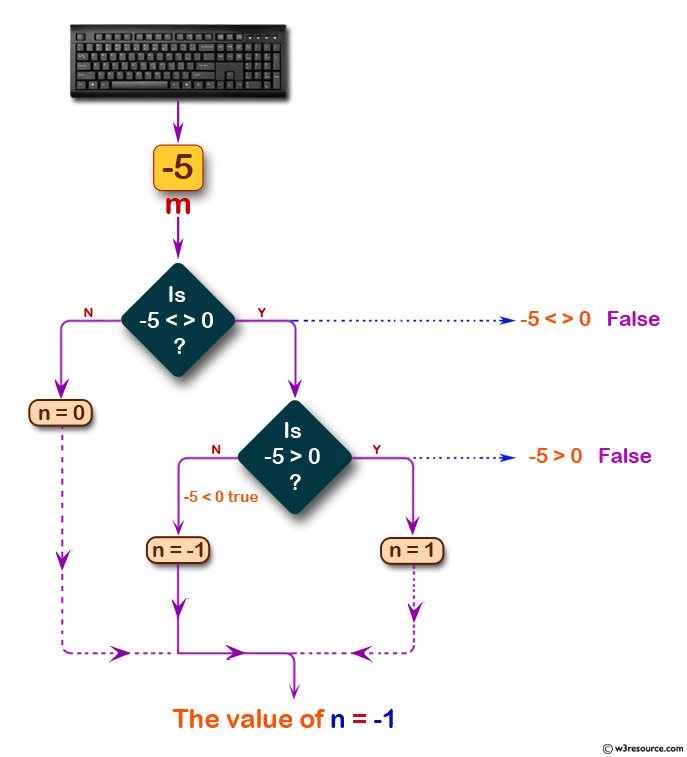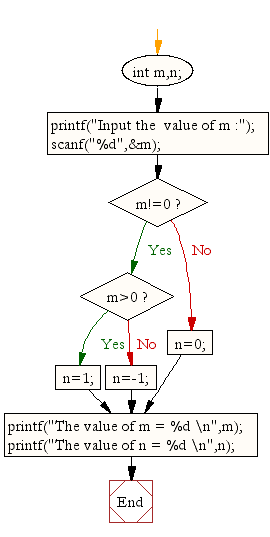﻿ C : Value of an integer n is 1,0 and -1 for the value of m# C Exercises: Display the value of an integer n is 1,0 and -1 for the value of an integer m

## C Conditional Statement: Exercise-6 with Solution

Write a C program to read the value of an integer m and display the value of n is 1 when m is larger than 0, 0 when m is 0 and -1 when m is less than 0.

Pictorial Presentation:Sample Solution:

C Code:

``````#include <stdio.h>
void main()
{
int m,n;
printf("Input the  value of m :");
scanf("%d",&m);
if(m!=0)
if(m>0)
n=1;
else
n=-1;
else
n=0;
printf("The value of m = %d \n",m);
printf("The value of n = %d \n",n);
}
```
```

Sample Output:

```Input the  value of m :-5
The value of m = -5
The value of n = -1
```

Flowchart:C Programming Code Editor:

Improve this sample solution and post your code through Disqus.

What is the difficulty level of this exercise?

Test your Programming skills with w3resource's quiz.

﻿

## C Programming: Tips of the Day

Why do C and C++ compilers allow array lengths in function signatures when they're never enforced?

It is a quirk of the syntax for passing arrays to functions.

Actually it is not possible to pass an array in C. If you write syntax that looks like it should pass the array, what actually happens is that a pointer to the first element of the array is passed instead.

Since the pointer does not include any length information, the contents of your [] in the function formal parameter list are actually ignored.

Ref : https://bit.ly/3fhlvdH